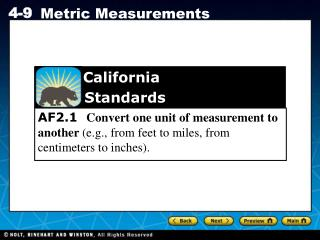DownloadDownload PresentationCalifornia Standards

# California Standards

Télécharger la présentation## California Standards

- - - - - - - - - - - - - - - - - - - - - - - - - - - E N D - - - - - - - - - - - - - - - - - - - - - - - - - - -
##### Presentation Transcript

1. California Standards AF2.1 Convert one unit of measurement to another (e.g., from feet to miles, from centimeters to inches).

2. You can use the following benchmarks to help you understand millimeters, grams, and other metric units. Write this at the top of your paper

3. Example 1: Choosing the Appropriate Metric Unit Choose the most appropriate metric unit for each measurement. Justify your answer. A. The amount of water a runner drinks each day Liters—The amount of water a runner drinks each day is similar to the amount of water in a large water bottle. B. The length of a boat Meters—The length of a boat is similar to the length of several doorways. C. The mass of a car Kilograms—The mass of a car is similar to the mass of several hundred textbooks.

4. The prefixes of metric units correlate to place values in the base-10 number system. The table shows how metric units are based on powers of 10. Write this at the bottom of page 103. You can convert units within the metric system by multiplying or dividing by powers of 10. To convert to a smaller unit, you must multiply. To convert to a larger unit, you must divide.

5. Example 2A: Converting Metric Units Convert the measure. 530 cL to liters 100 cL = 1L, so divide by 100. 530 cL = (530 ÷ 100) L Move the decimal point 2 places left: 5.30. = 5.3 L

6. Example 2B: Converting Metric Units Convert the measure. 1,070 g to milligrams 1 g = 1000 mg, so multiply by 1000. 1,070 g = (1,070 1,000) mg Move the decimal point 3 places right: 1,070.000. = 1,070,000 mg

7. Example 3: Using Unit Conversion to Make Comparisons Eliza buys one pumpkin that has a mass of 3 kg and another that that has a mass of 2,150 g. Which pumpkin has the greater mass? Use estimation to explain why your answer makes sense. Convert 2,150 g to kilograms. 1,000 g = 1 kg, so divide by 1,000. 2,150 g = (2,150 ÷1,000) kg = 2.150kg Move the decimal point 3 places to the left. The 3 kg pumpkin has the greater mass. Check Round 2,150 to the nearest thousand. 2,150 g is about 2,000 g or 2 kg 3 kg > 2 kg, so the answer makes sense.

8. Check It Out! Example 1 Choose the most appropriate metric unit for the measurement. Justify your answer. The amount of liquid in 10 teardrops Milliliters—The amount of liquid in 10 teardrops is similar to the amount of liquid in several eyedroppers.

9. Check It Out! Example 2 Convert the measure. 580 g to centigrams 1 g = 100 cg, so multiply by 100. 580 g = (580  100) cg Move the decimal point 2 places right: 580.00. = 58,000 cg

10. Check It Out! Example 3 Tyesha purchases a bag of potatoes that has a mass of 2.5 kg and Casey purchases a bag with a mass of 3,850 g. Which bag of potatoes has the greater mass? You can convert the mass of Tyesha’s bag to grams. 1 kg = 1000 g, so multiply by 1,000. 2.5 kg = (2.5  1,000) g Move the decimal point 3 places right: 2.500. = 2,500 g Tyesha’s 2,500 g bag of potatoes has a smaller mass.

11. Check It Out! Example 3 Continued Tyesha purchases a bag of potatoes that has a mass of 2.5 kg and Casey purchases a bag with a mass of 3,850 g. Which bag of potatoes has the greater mass? Check 3,850 g is about 4000 g or 4 kg. 4 kg > 2.5 kg, so the answer is reasonable.